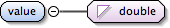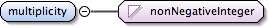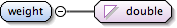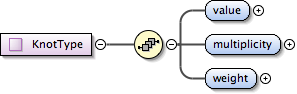### Showing:

 Annotations Diagrams Properties Source Used by
Element gml:KnotType / gml:value
Namespace http://www.opengis.net/gml
Annotations
 `The property "value" is the value of the parameter at the knot of the spline. The sequence of knots shall be a non-decreasing sequence. That is, each knot's value in the sequence shall be equal to or greater than the previous knot's value. The use of equal consecutive knots is normally handled using the multiplicity.`
DiagramType double
Properties
 content: simple
Source
 ``` The property "value" is the value of the parameter at the knot of the spline. The sequence of knots shall be a non-decreasing sequence. That is, each knot's value in the sequence shall be equal to or greater than the previous knot's value. The use of equal consecutive knots is normally handled using the multiplicity. ```
Schema location http://schemas.opengis.net/gml/3.1.1/base/geometryPrimitives.xsd
Element gml:KnotType / gml:multiplicity
Namespace http://www.opengis.net/gml
Annotations
 `The property "multiplicity" is the multiplicity of this knot used in the definition of the spline (with the same weight).`
DiagramType nonNegativeInteger
Properties
 content: simple
Source
 ``` The property "multiplicity" is the multiplicity of this knot used in the definition of the spline (with the same weight). ```
Schema location http://schemas.opengis.net/gml/3.1.1/base/geometryPrimitives.xsd
Element gml:KnotType / gml:weight
Namespace http://www.opengis.net/gml
Annotations
 `The property "weight" is the value of the averaging weight used for this knot of the spline.`
DiagramType double
Properties
 content: simple
Source
 ``` The property "weight" is the value of the averaging weight used for this knot of the spline. ```
Schema location http://schemas.opengis.net/gml/3.1.1/base/geometryPrimitives.xsd
Complex Type gml:KnotType
Namespace http://www.opengis.net/gml
Annotations
 `A knot is a breakpoint on a piecewise spline curve.`
DiagramUsed by
 Element gml:KnotPropertyType/gml:Knot
Model gml:value , gml:multiplicity , gml:weight
Children gml:multiplicity, gml:value, gml:weight
Source
 ``` A knot is a breakpoint on a piecewise spline curve. The property "value" is the value of the parameter at the knot of the spline. The sequence of knots shall be a non-decreasing sequence. That is, each knot's value in the sequence shall be equal to or greater than the previous knot's value. The use of equal consecutive knots is normally handled using the multiplicity. The property "multiplicity" is the multiplicity of this knot used in the definition of the spline (with the same weight). The property "weight" is the value of the averaging weight used for this knot of the spline. ```
Schema location http://schemas.opengis.net/gml/3.1.1/base/geometryPrimitives.xsd